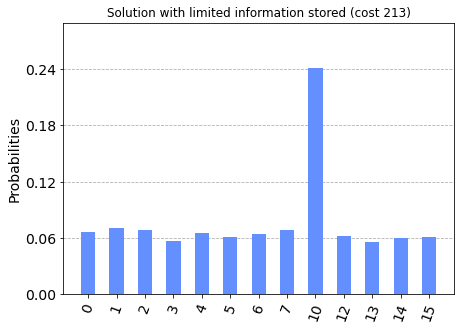# A note on the winning solution of the Fall 2020 IBM Quantum Challenge

After a nearly two-week-long wait, we finally got to see the winning solution of the Fall 2020 IBM Quantum Challenge. Below, we present our initial thoughts on what we were able to observe.

First, let us recall the following fragment of the contest rules.

"When mapping the board information into your quantum circuit, you must not change the board information from the original one."

The winning solution does not map the full board information into the quantum circuit. Instead, it only classically checks whether 24 specific patterns of stars occur on each of the boards. It is worth noting the following two facts about such an approach:

• It is lossy i.e. it is impossible to recover the board information from the data mapped into the quantum circuit.
• It constitutes a significant classical step towards solving the problem. The only remaining work to be done by the circuit is to check for each board whether any of the 24 positions is marked, with the knowledge that this occurs for only one board (and no more than two positions are marked).

It is completely up to the organizers what consitutes "changing the board information from the original one". However, looking at the contests's Slack channel, it seems that most of the competitors think that such a change is going too far.

Let us leave the discussion if such an approach violates the rules or not, and instead let us see how small the solution circuit can be made using the same classical preprocessing. After all, that is what the contest was all about!

We applied the optimizations from our solutions (see Adam's and Witek's write-ups), while preserving the amount of data transmitted. The cost of the resulting circuit is 213. Enjoy reading the code!

## New concept of the buffer

Recall that the unsolvable board has at most one odd and at most one even permutation marked. Therefore, instead of storing the full bitmap of 24 permutations, it is enough to store two indices – one for the (potential) odd permutation and one for the (potential) even permutation. We use a data buffer in the following way: `qbuf[0:4]` stores the id of the even permutation and `qbuf[4:8]` – the odd one. Zero is treated as no permutation appearing.

To indicate the id of the buffer associated with a particular board we assign the buffer slot in `qbufid` variable (0 – none, 1 – the first buffer). Observe that one bit is sufficient as we are guaranteed that only one of the boards is unsolvable i.e. no more than one buffer will be allocated.

``````def obtain4_many_cp(qc, inv, boards, qbid, qbidanc, qbuf, qbufid):
qctrl = qbidanc

# We are guaranteed that only one board is unsolvable,
# so we will heep the `qctrl` bit marked by performing explicit
def open_ctrl(bid):
mrg(qc, qbid, qbid, qbidanc,
mask=(not (bid & 0b0001), not (bid & 0b0010)))
mrg(qc, qbid, qbid, qbidanc,
mask=(not (bid & 0b0100), not (bid & 0b1000)))
mrg(qc, qbidanc, qbidanc, qbidanc)

def close_ctrl(bid):
mrg(qc, qbidanc, qbidanc, qbidanc)
mrg(qc, qbid, qbid, qbidanc,
mask=(not (bid & 0b0100), not (bid & 0b1000)))
# Not uncomputing the following in small diffuser setting
# mrg(qc, qbid, qbid, qbidanc,
#   mask=(not (bid & 0b0001), not (bid & 0b0010)))

opened_bid = None

for bid in range(len(boards)):
for perm_id, p in enumerate(permutations_even()):
for even in [True, False]:
if all(boards[bid][i, p[i]] for i in range(4)):
if opened_bid is None:
if not inv:
open_ctrl(bid)
opened_bid = bid
else:
assert bid == opened_bid

# Marking that the data associated
# with this board is in 1st buffer.
qc.cx(qctrl, qbufid)

# The permutation id stored in the buffer
# (for even/odd permutations)
qsubbuf = [qbuf[0:4], qbuf[4:8]][even]
if (perm_id+1) & 0b0001:
qc.x(qsubbuf)
if (perm_id+1) & 0b0010:
qc.x(qsubbuf)
if (perm_id+1) & 0b0100:
qc.x(qsubbuf)
if (perm_id+1) & 0b1000:
qc.x(qsubbuf)

# Transform even permutation into odd one
p = tuple([1, 0, 2, 3][p[i]] for i in range(4))

if opened_bid is not None:
if inv:
close_ctrl(opened_bid)
``````
``````def permutations_even():
return [
(0, 1, 2, 3),
(0, 2, 3, 1),
(0, 3, 1, 2),
(1, 0, 3, 2),
(1, 2, 0, 3),
(1, 3, 2, 0),
(2, 0, 1, 3),
(2, 1, 3, 0),
(2, 3, 0, 1),
(3, 0, 2, 1),
(3, 1, 0, 2),
(3, 2, 1, 0),
]
``````
``````def oracle_cp(qc, boards, qbid, qbuf, qbufid, qbidanc, qanc):
obtain4_many_cp(qc, False, boards, qbid, qbidanc, qbuf, qbufid)
mark_unsolvable_cp(qc, qbuf, qbufid, qanc)
obtain4_many_cp(qc, True, boards, qbid, qbidanc, qbuf, qbufid)
``````

Observe that `qbufid` has only one bit, therefore `qbufid != 0` is equivalent to `qbufid == 1`, which implies that there is some permutation stored in the buffer associated with this board.

``````def mark_unsolvable_cp(qc, qbuf, qbufid, qanc):
qc.z(qbufid)
``````
``````def diffusion2(qc, qbits):
assert len(qbits) == 2

hx(qc, qbits)
qc.z(qbits) #HXH
qc.cx(qbits, qbits)
qc.z(qbits) #HXH
xh(qc, qbits)
``````
``````def hx(qc, q):
qc.u3(pi/2, 0, 0, q)

def xh(qc, q):
qc.u3(-pi/2, 0, 0, q)
``````
``````def solution_cp(problem_set):
boards = [read_board(board_spec) for board_spec in problem_set]

# Board id [0 - MSB, 3 - LSB]
qbid = QuantumRegister(4, name='bid')

# Buffer for the lossely compressed board info
qbuf = QuantumRegister(8, name='buf')

qbufid = QuantumRegister(1, name='bufid')

qbidanc = QuantumRegister(3, name='bidanc')

# General-purpose ancillae
qanc = QuantumRegister(3, name='anc')

# Measured answer (endianness as in `qbid`)
cans = ClassicalRegister(4, name='c')

qc = QuantumCircuit(
qbid, qbuf, qbufid,
qbidanc, qanc,
cans,
)

# Initial superposition
qc.h(qbid)

oracle_cp(qc, boards, qbid, qbuf, qbufid, qbidanc, qanc)

diffusion2(qc, [qbid, qbid])

qc.measure(qbid, cans)

return qc
``````
``````simulate(
solution_cp(problem_set),
title='Solution with limited information stored')
``````